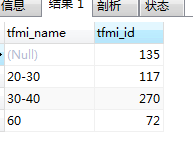SQl数据取的不完整是什么问题

SQL语句 结果显示的不完整
SELECT
age AS 'tfmi_name',
count( * ) AS 'tfmi_id'
FROM
(
SELECT
CASE

WHEN
TIMESTAMPDIFF( YEAR, tcce_birthday, CURDATE( ) ) >= 0
AND TIMESTAMPDIFF( YEAR, tcce_birthday, CURDATE( ) ) <= 10 THEN '0-10' WHEN TIMESTAMPDIFF( YEAR, tcce_birthday, CURDATE( ) ) >= 10
AND TIMESTAMPDIFF( YEAR, tcce_birthday, CURDATE( ) ) <= 20 THEN '10-20' WHEN TIMESTAMPDIFF( YEAR, tcce_birthday, CURDATE( ) ) >= 20
AND TIMESTAMPDIFF( YEAR, tcce_birthday, CURDATE( ) ) <= 30 THEN '20-30' WHEN TIMESTAMPDIFF( YEAR, tcce_birthday, CURDATE( ) ) >= 30
AND TIMESTAMPDIFF( YEAR, tcce_birthday, CURDATE( ) ) <= 40 THEN '30-40' WHEN TIMESTAMPDIFF( YEAR, tcce_birthday, CURDATE( ) ) >= 40
AND TIMESTAMPDIFF( YEAR, tcce_birthday, CURDATE( ) ) <= 40 THEN '40-50' WHEN TIMESTAMPDIFF( YEAR, tcce_birthday, CURDATE( ) ) >= 50
AND TIMESTAMPDIFF( YEAR, tcce_birthday, CURDATE( ) ) <= 40 THEN '50-60' WHEN TIMESTAMPDIFF( YEAR, tcce_birthday, CURDATE( ) ) >= 60 THEN
'60'
END AS age,
tfmi_id
FROM
tb_family_member_info
LEFT JOIN tb_building_room ON tbr_id = tfmi_room_id
LEFT JOIN tb_building_info ON tbr_building_id = tbi_id
LEFT JOIN tb_grid_community_refer ON tgcr_buildiing_id = tbi_id
LEFT JOIN tb_community_unit_info ON tgcr_unit_id = tcui_id
LEFT JOIN tb_community_info ON tci_id = tci_id
LEFT JOIN tb_grid_info ON tgcr_grid_id = tgi_id
LEFT JOIN tb_community_center_info ON tgi_community_center_id = tgi_community_center_id
WHERE
tfmi_status <> 0
AND tgcr_status = 1
AND tcci_status = 1
AND tgi_status = 1
AND tic_status = 1
AND tbi_status = 1
AND tcui_status = 1
AND tbr_status = 1
) a
GROUP BY
age

2个回答